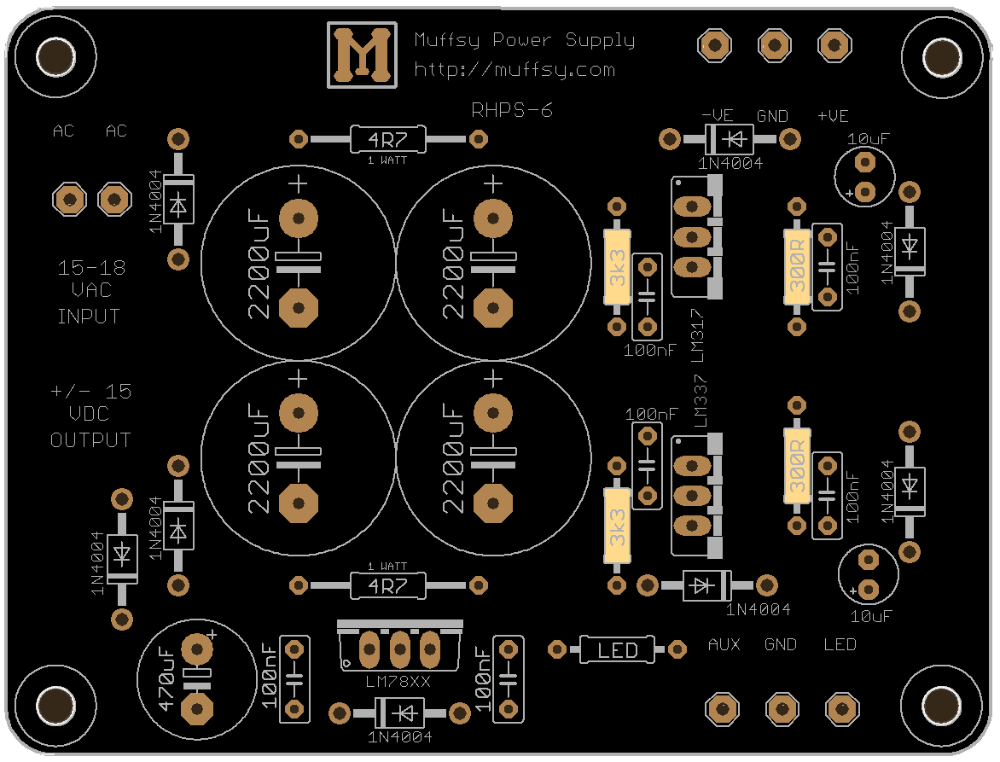# Muffsy Phono Kits

## Change the Output Voltage of the Muffsy Hifi Power Supply

The output voltage of the Muffsy Hifi Dual Power Supply can be changed to suit your needs. Let's have a look at how this can be done, and how to make sure that your transformer is able to deliver sufficient voltage.

Here's the layout of the printed circuit board. There are only two resistors that need to be replaced to change the output voltage, and they are shown here in yellow (3k3):Resistors that control the output voltage

With the resistors at 3300 ohms, the power supply will deliver +/-15 volts DC.

## Resistor Values

You might want less than +/- 15 volts. Let's say +/-12.5 volts. Or more, for instance +/-17.5 volts. These output values are well within reach using the recommended 15-18VAC wall adapter.

This table will give you the resistor values for 12.5, 15 and 17.5 volts dual output:

Resistor, ohms Output voltage, +/- volts DC
2.700 (2k7)
12.5
3.300 (3k3) 15.0
3.900 (3k9) 17.5

Other output voltages are easily obtainable, just use a calculator such as this one.

The R1 value to provide in the calculator is 300 ohm.

## Wall Adapter Voltage

The PSU needs a higher input value than the desired output value. Then how can a 15 volt AC-adapter be sufficient for 17.5V output?

The answer to this is that the rectified DC voltage will be higher than the AC input voltage by a factor of 1.41. Now, there is some loss in the diodes. To be on the safe side, we'll use the factor 1.3 instead:

• 15V * 1.3 = 19.5V

This is more than 17.5 volts, and you are in the clear!

So what is the lowest AC voltage needed for a 15V supply?

• 15V / 1.3 = 11.54V

You could actually use a 12V AC-adapter. But, there is the possibility that an adapter rated at 12V will give less than that. Stick with 15V or more, and you can be sure that your PSU works as expected.

Desktop Site